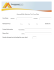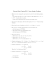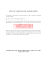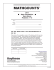# Exercises 09

## Transcription

Exercises 09
```METU
DEPARTMENT OF MATHEMATICS
Math 112 Discrete Mathematics
Exercise 9
1) Let  = {1,2,3,4,5,6,7,8}.
a) If five integers are selected from A, must at least one pair of integers have a sum 9?
b) If four integers are selected from A, must at least one pair of integers have a sum 9?
2) Given a group of  women and their husbands, how many people must be chosen from this
group of 2 people in order to guarantee that the set of those selected contains a married
couple
3) In a round-robin tournament (in which every player plays against every other player exactly
once), suppose that each player wins at least once. Show that there are at least two players
with the same number of wins
4) To guarantee that there are ten diplomats from the same continent at a party, how many
diplomats must be invited if they are chosen from
a) 12 Australian, 14 African, 15 Asian, 16 European, 18 South American, and 20 North
American diplomats.
b) 7 Australian, 14 African, 8 Asian, 16 European, 18 South American, and 20 North American
diplomats.
5) Show that, in a group of 150 people, at least six must have the same last initial.
6) There are 42 students who are to share 12 computers. Each student uses exactly one
computer and no computer is used by more than 6 students. Show that at least five
computers are used by three or more students.
7) A bag contains exactly 6 red, 5 white, and 7 marbles. Find the least number of marbles to be
picked which will ensure that either at least 3 red or at least 4 white or at least 5 blue
marbles picked.
8) In any set of 1001 integers chosen from {1,2,3, … ,2000}, show that there must be two
members such that one is divisible by the other.
9) Suppose that the numbers 1,2, … ,100 are randomly placed in 100 locations on a circle. Show
that there exist three consecutive locations so that the sum of integers at these locations is at
least 152.
10) A violinist practiced for a total of 110 hours over a period of 12 days. Show that he practiced
at least 19 hours on some pair of consecutive days. Assume that he practiced a whole number
of hours on each day.
11) Prove that among any five points selected inside an equilateral triangle with side equal to 1,
1
there always exists a pair at the distance not greater than 2.
12) Each of the given 9 lines cuts a given square into two quadrilaterals whose areas are in
proportion 2: 3. Prove that at least three of these lines pass through the same point.
13) Five points are chosen at the nodes of a square lattice (grid). Why is it certain that at least
one mid-point of a line joining a pair of chosen points, is also a lattice point?
14) Suppose () is a polynomial with integral coefficients. If () = 2 for three different
integers , , and , prove that, for no integer, () can be equal to 3.
15) Prove that there exist two powers of 3 whose difference is divisible by 1997.
16) Prove that there exists a power of three that ends with 001.
17) If more than 500 integers from {1,2, … ,1000} are selected, then some two of the selected
integers have the property that one divides the other.
18) A person takes at least one aspirin a day for 30 days. If he takes 45 aspirin altogether, in some
sequence of consecutive days he takes exactly 14 aspirin.
19) A theater club gives 7 plays one season. Five women in the club are each cast in 3 of the plays.
Then some play has at least 3 women in its cast.
20) Prove that at any party of  people, some pair of people are friends with the same number of
people at the party.
21) Let  = {3,4,5,6,7,8,9,10,11,12,13}. Suppose six integers are chosen from . Must there be two
integers whose sum is 16?
22) Let  = {3,4,5,6,7,8,9,10,11,12,13}. Suppose seven integers are chosen from . Must there be
two integers whose sum is 16?
23) Let  = {7, 8, 9, … ,97}. At least how many integers should be chosen from S to guarantee the
existence of two integers whose sum is 100?
24) Let  be a set of six positive (distinct) integers each of which is less then 13. Show that there
must be two distinct subsets of  sums of whose elements are same.
25) During a campaign, a politician visits 45 towns in 30 days. If he visits a positive whole
number of towns each day, show that there must exist some period of consecutive days
during which he visits exactly 14 towns.
Solutions
1)
a) Observe that there are only four pairs of
integers in  that add up to 9, and that each
integer exactly occurs in exactly one pair. These
pairs are 1 + 8, 2 + 7, 3 + 6, 4 + 5. Let the
pigeons be the five integers selected from , and
let the pigeonholes be the pairs of integers that
add up to 9. According to pigeonhole principle,
since there are more pigeons (5) than pigeonholes
(4), at least two pigeons must go to the same hole.
Thus, two distinct integers are sent to the same
pair. But that implies that those two integers are
the two distinct elements of the pair, so their sum
is 9.
b) The answer is no. For instance consider the
numbers: 1, 2, 3, 4.
2) Think of associating the married couples with
boxes labeled 1 to , so that whenever a member
is selected from the set of 2 people, then that
person is placed into his or her associated box.
Thus the question reduces to asking for the
smallest number of members that can be placed in
the  boxes in order that some box contains two
members. Clearly  does not suffice; however, by
the pigeonhole principle,  + 1 works.
3) The number of wins for a player is 1 or 2 or 3 … or
− 1. These  − 1 numbers correspond to  − 1
pigeonholes in which the pigeons (players) are to
be housed. So at least two of them should be in the
same pigeonhole and they have the same number
of wins.
4)
a) 55
b) 52
5) In this example, the pigeons are the 150 people
and the pigeonholes are the 29 possible last
initials of their names (we consider the Turkish
alphabet which consists of 29 letters). Note that
150 > 5 × 28 = 140. Then, the generalized
pigeonhole principle states that some initial must
be the image of at least six (5 + 1) people. Thus at
least six people have the same last initial.
6) Suppose that only four computers were used by
three or more students. At most six students are
allowed to share any computer, making a total of
at most 24 students using these four computers.
Since there are 42 students at all, that would leave
at least 18 students to share the remaining eight
computers with no more than two students per
computer. But the generalized pigeonhole
principle guarantees that if 18 students share
eight computers, then at least three must share
one of them. This is a contradiction. Thus the
supposition is false, and so at least five computers
are used by three or more students.
7) The least number of marbles to be picked is
(3 − 1) + (4 − 1) + (5 − 1) + 1 = 10.
8) Let 1 , 2 , . . . , 1001 denote the 1001 integers
chosen. We can express each  ,  = 1,2, . . . ,1001
in the form  = 2  where  is odd (for
instance, 564 = 22 ⋅ 141, 1184 = 25 ⋅ 37, 512 =
29 9 ⋅ 1, 97 = 20 ⋅ 97). Now, consider the odd
integers 1 , 2 , … , 1001 . But, there are exactly
1000 odd integers between 1 and 2000, hence by
the pigeonhole principle, at least two of
1 , 2 , … , 1001 are equal. So  =  for some
and , where  ≠ . Then we have  = 2  and
= 2  . Since  ≠  , we have  ≠  ;
without loss of generality we can assume  <
. But then 2 |2 , and consequently,  | .
(Is it possible to choose 1000 integers from the list
so that no number is divisible by any other?)
9) Number the locations 1,2, … ,100 and let  be the
number assigned to location ,  = 1,2, … ,100.
There are 100 sums to consider: 1 + 2 +
3 , 2 + 3 + 4 , . . . , 98 + 99 + 100
,
99 +
100 + 1 , 100 + 1 + 2 and each  appears in
exactly three of the sums. Hence, the total of these
sums
is3(1 + 2 + ⋯ + 100 ) = 3 ⋅ 5050 =
15150. Thus, the average value of is 15150/100 =
151.5, and so by the previous problem, one of the
sums has value at least 152.
10) Number the days 1,2, … ,12 and consider the
subsets. {1,2}, {3,4}, {5,6}, {7,8}, {9,10}, {11,12}.
Since these subsets partition the 12 −day period,
the 110 hours of practice can be distributed
among them. And since 110 > 18 × 6 = 108, the
generalized pigeonhole principle implies that
some consecutive 2 −day period contains at least
19 hours.
11) Split the triangle into four smaller ones by
connecting midpoints of its sides. The largest
possible distance between two points of one small
triangle is ½. Now, we are given 4 triangles and 5
points. By the Pigeonhole Principle, at least one
triangle contains at least two points. The distance
between any two such points does not exceed ½.
12) None of the given lines may pass
through two successive sides of
the square because in this case
we get a triangle and a pentagon
Assume one of them intersects sides  and  at
points  and , respectively. The quadrilaterals,
and , are both trapezoids with equal
heights. Therefore, their areas are in the same
ratio as their midlines. From here,  intersects
the midline of the square in ratio 2:3. This is true
for any one of the nine lines. But there are only
four points that divide the midlines of the square
in the ratio 2:3. Therefore, by the Pigeonhole
Principle, at least three of the lines pass through
the same point.
17) Form the  consisting of the  −th odd integer less
than 2 together with its multiples by powers of
2:
1 = {1, 2, 4, , … ,512},
3 = {3, 6, 12, 24, … ,768},
5 = {5, 10, 20, … ,640 },
⋮
999 = {999}.
Then the union of these  sets contains
{1,2, … ,2}. Hence some two of the selected
integers belongs to  , for some ; and so one of
them divides the other.
13) The midpoint of the line joining two grid points
(1 , 1 ) and (2 , 2 ) is located at (
1 +2 1 +2
2
,
2
).
The latter will be a grid point iff its coordinates
are integers. The -coordinate will be integer iff 1
and 2 have the same parity, i.e., iff they are either
both even or both odd. Out of 5 points, at least
three satisify this condition. But the same is true
of the -coordinate. And out of the selected three
points, at least two have y-coordinate with the
same parity.
14) In the following we assume () is a polynomial
with integral coefficients:
() =    + −1  −1 + . . . + 1  + 0
Lemma. For any two different integers
and , the difference ()() is divisible
by .
18) Let  be the total number of aspirin consumed up
to and including the  −th day, for  = 1, … ,30.
Combine these with the numbers 1 +
14, . . . , 30 + 14, providing 60 numbers, all
positive and less or equal 45 + 14 = 59. Hence
two of these 60 numbers are identical. Since all
's and, hence, ( + 14)'s are distinct (at least
one aspirin a day consumed), then  =  + 14,
for some  < . Thus, on days  + 1 to , the person
consumes exactly 14 aspirin.
Proof. Indeed, ()() =  (  ) +
−1 (−1 −1 ) + . . . + 1 ( − ) and,
since
() | (  ) for every integer  > 0.
19) Five women each cast in 3 plays makes 15
Now, assume that () = () = () = 2 and
() = 3 with all , ,  and  different. From
Lemma we immediately obtain that
20) A person at the party can have 0 up to  − 1
friends at the party. However, if someone has 0
friends at the party, then no one at the party has
− 1 friends at the party, and if someone has  −
1 friends at the party, then no one has 0 friends at
the party. Hence the number of possibilities for
the number of friends the  people at the party
have must be less than n. Hence two people at the
party have the same number of friends at the
party.
() | (()()) = 32 = 1,
() | (()()) = 32 = 1,
() | (()()) = 32 = 1.
Thus differences , ,  all divide 1. But 1
has only two divisors: 1 and −1. Therefore, by the
Pigeonhole Principle, two of the differences
coincide. Which contradicts our assumption that
the numbers a, b, c are all different.
15) There are 1997 remainders of division by 1997.
Consider the sequence of powers 1,3,32 , … , 31997 .
It contains 1998 members. Therefore, by the
Pigeonhole principle, some two of them, say 3
and 3,  > , have equal remainders when
divided by 1997. Then their difference (33) is
divisible by 1997.
16) As in previos problem, let 3 and 3 ( > )
have the same remainder when divided by 1000.
Thus 3 3 = 3 (3− 1) is divisible by 1000.
Since 1000 and 3 have no common factors, 1000
is bound to divide the second factor (3− 1).
This exactly means that 3− ends with 001.
woman's parts in the 7 plays. Since
15
7
> 2, some
play has at least 3 women in its cast.
21) No. Consider the set {3,4,5,6,7,8}
22) Yes. Any subset with 7 or more elements must
contain both members of at least one of the
following pairs:
(3,13), (4,12), (5,11), (6,10), (7,9)
23)
Consider the partition:
(7,93), (8,92), … , (49,51) , (50),
⏟
⏟ (94), (95), (96), (97)
43
5−
24)  has 64 subsets and largest possible sum is 7 +
8 + 9 + 10 + 11 + 12 = 57.
25) See Problem 10.
Problems and solutions are taken from the site: www.cut-theknot.org
```

#### Similar documents### Document 6487473### Discrete Math, Spring 2013 - Some Sample Problems### The Paperless Math Classroom Brian Aspinall Teacher, LKDSB### Sample Individual Questions 2012 Canadian Team Mathematics Contest (CTMC)